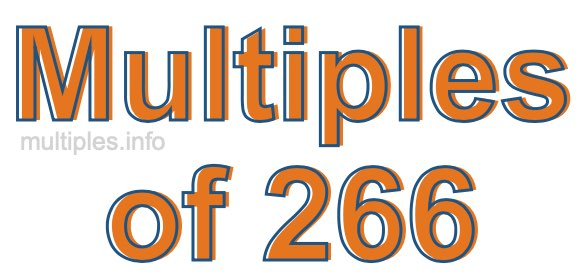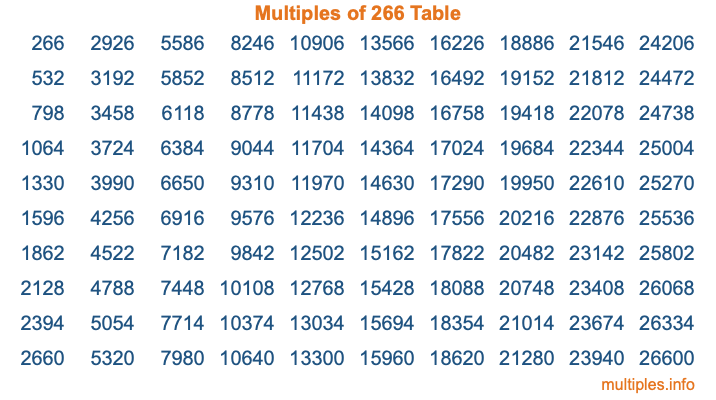Multiples of 266Welcome to the Multiples of 266 page. Here we will first teach you everything you will ever need to know about the multiples of 266, and then give you a study guide summary of everything we taught you to make sure you remember it all. Use this page to look up facts and learn information about the multiples of 266. This page will make you a multiples of two hundred sixty-six expert!

Definition of Multiples of 266
Multiples of 266 are all the numbers that when divided by 266 equal an integer. Each of the multiples of 266 are called a multiple. A multiple of 266 is created by multiplying 266 by an integer.

Therefore, to create a list of multiples of 266, you start with 1 multiplied by 266, then 2 multiplied by 266, then 3 multiplied by 266, and so on for as long as you want. Thus, the list of the first five multiples of 266 is 266, 532, 798, 1064, and 1330. To see a larger list of multiples of 266, see the printable image of Multiples of 266 further down on this page. We also have a category where you can choose any nth multiple of 266.

Multiples of 266 Checker
The Multiples of 266 Checker below checks to see if any number of your choice is a multiple of 266. In other words, it checks to see if there is any number (integer) that when multiplied by 266 will equal your number. To do that, we divide your number by 266. If the the quotient is an integer, then your number is a multiple of 266.

Is  a multiple of 266?

Least Common Multiple of 266 and ...
A Least Common Multiple (LCM) is the lowest multiple that two or more numbers have in common. This is also called the smallest common multiple or lowest common multiple and is useful to know when you are adding our subtracting fractions. Enter one or more numbers below (266 is already entered) to find the LCM.

Check out our LCM Calculator if you need more details about the Least Common Multiple or if you need the LCM for different numbers for adding and subtraction fractions.

nth Multiple of 266
As we stated above, 266 is the first multiple of 266, 532 is the second multiple of 266, 798 is the third multiple of 266, and so on. Enter a number below to find the nth multiple of 266.

th multiple of 266

Multiples of 266 vs Factors of 266
266 is a multiple of 266 and a factor of 266, but that is where the similarities end. All postive multiples of 266 are 266 or greater than 266. All positive factors of 266 are 266 or less than 266.

Below is the beginning list of multiples of 266 and the factors of 266 so you can compare:

Multiples of 266: 266, 532, 798, 1064, 1330, etc.

Factors of 266: 1, 2, 7, 14, 19, 38, 133, 266

As you can see, the multiples of 266 are all the numbers that you can divide by 266 to get a whole number. The factors of 266, on the other hand, are all the whole numbers that you can multiply by another whole number to get 266.

It's also interesting to note that if a number (x) is a factor of 266, then 266 will also be a multiple of that number (x).

Multiples of 266 vs Divisors of 266
The divisors of 266 are all the integers that 266 can be divided by evenly. Below is a list of the divisors of 266.

Divisors of 266: 1, 2, 7, 14, 19, 38, 133, 266

The interesting thing to note here is that if you take any multiple of 266 and divide it by a divisor of 266, you will see that the quotient is an integer.

Multiples of 266 Table
Below is an image of the first 100 multiples of 266 in a table. The table is in chronological order, column by column. The first column has the first ten multiples of 266, the second column has the next ten multiples of 266, and so on.The Multiples of 266 Table is also referred to as the 266 Times Table or Times Table of 266. You are welcome to print out our table for your studies.

Negative Multiples of 266
Although not often discussed or needed in math, it is worth mentioning that you can make a list of negative multiples of 266 by multiplying 266 by -1, then by -2, then by -3, and so on, to get the following list of negative multiples of 266:

-266, -532, -798, -1064, -1330, etc.

Multiples of 266 Summary
Below is a summary of important Multiples of 266 facts that we have discussed on this page. To retain the knowledge on this page, we recommend that you read through the summary and explain to yourself or a study partner why they hold true.

There are an infinite number of multiples of 266.

A multiple of 266 divided by 266 will equal a whole number.

266 divided by a factor of 266 equals a divisor of 266.

The nth multiple of 266 is n times 266.

The largest factor of 266 is equal to the first positive multiple of 266.

266 is a multiple of every factor of 266.

266 is a multiple of 266.

A multiple of 266 divided by a divisor of 266 equals an integer.

266 divided by a divisor of 266 equals a factor of 266.

Any integer times 266 will equal a multiple of 266.

Multiples of a Number
Here you can get the multiples of another number, all with the same attention to detail as we did for multiples of 266 on this page.

Multiples of
Multiples of 267
Did you find our page about multiples of two hundred sixty-six educational? Do you want more knowledge? Check out the multiples of the next number on our list!